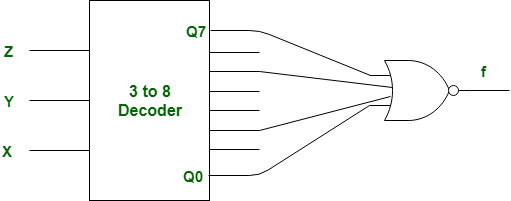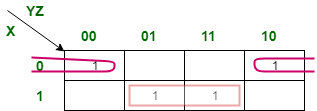Related Articles

# GATE | Gate IT 2008 | Question 9

• Last Updated : 20 Aug, 2019

What Boolean function does the circuit below realize ?(A) xz+x’z’
(B) xz’+x’z
(C) x’y’+yz
(D) xy+y’z’

Explanation: There 0, 2, 5, 7 are enable input in given decoder.

Therefore, given K-Map should be as following:So, output of above K-Map is,

```= xz + x'z'
= (x⊙z)
= x (ex-nor) z ```

But, there given gate is NOR instead of OR, therefore above output will be negatated.
So, output function f is,

```f = (xz + x'z')'
= x'z + xz'
= (x⊕z)
= x (xor) z ```

So, option (B) is correct.

This explanation is contributed by Deep Shah.

Quiz of this Question

Attention reader! Don’t stop learning now.  Practice GATE exam well before the actual exam with the subject-wise and overall quizzes available in GATE Test Series Course.

Learn all GATE CS concepts with Free Live Classes on our youtube channel.

My Personal Notes arrow_drop_up